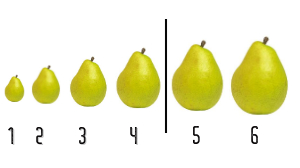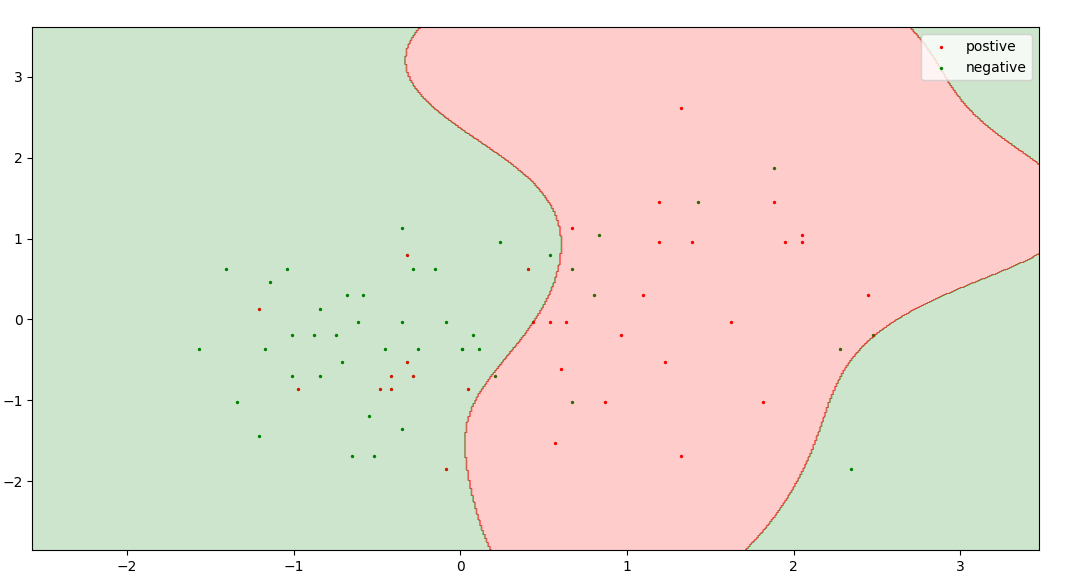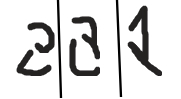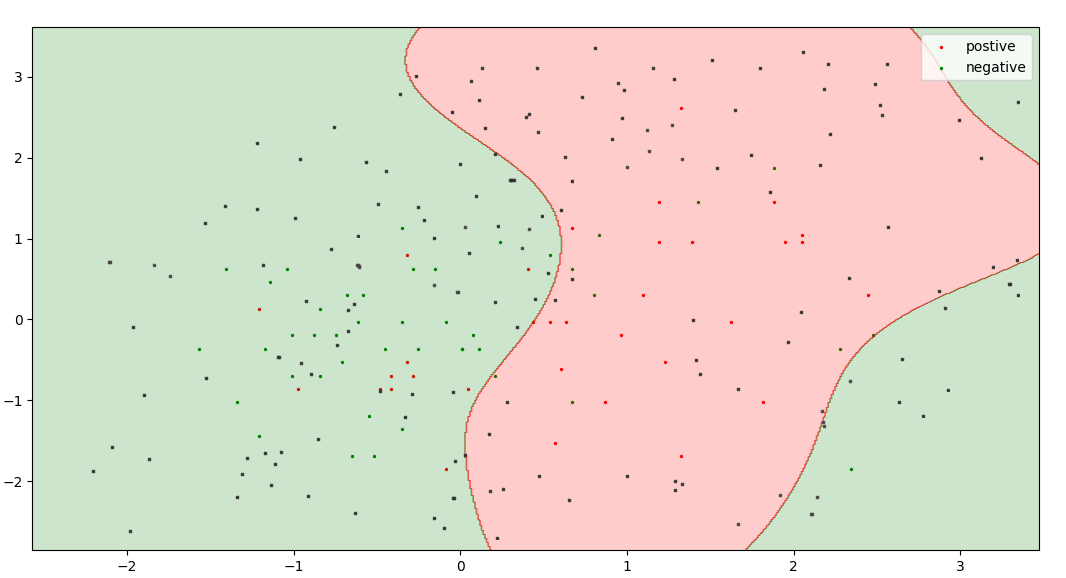# ML | Active Learning

What is Active Learning?
Active Learning is a special case of Supervised Machine Learning. This approach is used to construct a high performance classifier while keeping the size of the training dataset to a minimum by actively selecting the valuable data points.

Where should we apply active learning?

1. We have a very small amount or a huge amount of dataset.
2. Annotation of the unlabeled dataset cost human effort, time and money.

Example

On a certain planet, there are various fruits of different size(1-5), some of them are poisonous and others don’t. The only criteria to decide a fruit is poisonous or not is it’s size. our task is to train a classifier which predicts the given fruit is poisonous or not. The only information we have, is fruit with size 1 is not poisonous, the fruit of size 5 is poisonous and after a particular size, all fruits are poisonous.The first approach is to check each and every size of the fruit, which consume time and resources.
The second approach is to apply the binary search and find the transition point (decision boundary). This approach uses fewer data and gives the same results as of linear search.

``` General Algorithm :

1. train classifier with the initial training dataset
2. calculate the accuracy
3. while(accuracy < desired accuracy):
4.    select the most valuable data points (in general points close to decision boundary)
5.    query that data point/s (ask for a label) from human oracle
6.    add that data point/s to our initial training dataset
7.    re-train the model
8.    re-calculate the accuracy

```

Approaches Active Learning Algorithm
1. Query Synthesis

• Generally this approach is used when we have a very small dataset.
• This approach we choose any uncertain point from given n-dimensional space. we don’t care about the existence of that point.
•In this querry synthesis can pick any point(valuable) from 3*3 2-D plane.

• Sometime it would be difficult for human oracle to annotate the queried data point.
•These are some queries generated by Query Synthesis approach for a model trained for handwritten recognition. It is very difficult to annotate these queries.

2. Sampling

• This approach is used when we have a large dataset.
• In this approach we split our dataset into three parts: Training Set; Test Set; Unlabeled Pool(ironical) [5%; 25%, 70%].
• This trainig dataset is our initial dataset and is used to initially train our model.
• This approach selects valuable/uncertain points from this unlabeled pool, this ensures that all the querry can be recognized by human oracle
•Black points represents unlabeled pool and union red, green colour dots represents training dataset.

Here is an active learning model which decide valuable points on the basis of, the probability of a point present in a class. In Logistic Regression points closest to the threshold (i.e. probability = 0.5) is the most uncertain point. So, I choose the probability between 0.47 to 0.53 as a range of uncertainty.

 `import` `numpy as np ` `import` `pandas as pd ` `from` `statistics ``import` `mean ` `from` `sklearn.impute ``import` `SimpleImputer ` `from` `sklearn.preprocessing ``import` `StandardScaler ` `from` `sklearn.linear_model ``import` `LogisticRegression ` `from` `sklearn.model_selection ``import` `train_test_split ` ` `  ` `  `# split dataset into test set, train set and unlabel pool ` `def` `split(dataset, train_size, test_size): ` `    ``x ``=` `dataset[:, :``-``1``] ` `    ``y ``=` `dataset[:, ``-``1``] ` `    ``x_train, x_pool, y_train, y_pool ``=` `train_test_split( ` `        ``x, y, train_size ``=` `train_size) ` `    ``unlabel, x_test, label, y_test ``=` `train_test_split( ` `        ``x_pool, y_pool, test_size ``=` `test_size) ` `    ``return` `x_train, y_train, x_test, y_test, unlabel, label ` ` `  ` `  `if` `__name__ ``=``=` `'__main__'``: ` `    ``# read dataset ` `    ``dataset ``=` `pd.read_csv(``"./spambase.csv"``).values[:, ] ` ` `  `    ``# imputing missing data ` `    ``imputer ``=` `SimpleImputer(missing_values ``=` `0``, strategy ``=``"mean"``) ` `    ``imputer ``=` `imputer.fit(dataset[:, :``-``1``]) ` `    ``dataset[:, :``-``1``] ``=` `imputer.transform(dataset[:, :``-``1``]) ` ` `  `    ``# feature scalling ` `    ``sc ``=` `StandardScaler() ` `    ``dataset[:, :``-``1``] ``=` `sc.fit_transform(dataset[:, :``-``1``]) ` ` `  `    ``# run both models 100 times and take the average of their accuracy ` `    ``ac1, ac2 ``=` `[], []  ``# arrays to store accuracy of different models ` ` `  `    ``for` `i ``in` `range``(``100``): ` `        ``# split dataset into train(5 %), test(25 %), unlabel(70 %) ` `        ``x_train, y_train, x_test, y_test, unlabel, label ``=` `split( ` `            ``dataset, ``0.05``, ``0.25``) ` ` `  `        ``# train model by active learning ` `        ``for` `i ``in` `range``(``5``): ` `            ``classifier1 ``=` `LogisticRegression() ` `            ``classifier1.fit(x_train, y_train) ` `            ``y_probab ``=` `classifier1.predict_proba(unlabel)[:, ``0``] ` `            ``p ``=` `0.47` `# range of uncertanity 0.47 to 0.53 ` `            ``uncrt_pt_ind ``=` `[] ` `            ``for` `i ``in` `range``(unlabel.shape[``0``]): ` `                ``if``(y_probab[i] >``=` `p ``and` `y_probab[i] <``=` `1``-``p): ` `                    ``uncrt_pt_ind.append(i) ` `            ``x_train ``=` `np.append(unlabel[uncrt_pt_ind, :], x_train, axis ``=` `0``) ` `            ``y_train ``=` `np.append(label[uncrt_pt_ind], y_train) ` `            ``unlabel ``=` `np.delete(unlabel, uncrt_pt_ind, axis ``=` `0``) ` `            ``label ``=` `np.delete(label, uncrt_pt_ind) ` `        ``classifier2 ``=` `LogisticRegression() ` `        ``classifier2.fit(x_train, y_train) ` `        ``ac1.append(classifier2.score(x_test, y_test)) ` ` `  `        ``''' split dataset into train(same as generated by our model), ` `        ``test(25 %), unlabel(rest) '''` `        ``train_size ``=` `x_train.shape[``0``]``/``dataset.shape[``0``] ` `        ``x_train, y_train, x_test, y_test, unlabel, label ``=` `split( ` `            ``dataset, train_size, ``0.25``) ` ` `  `        ``# train model without active learning ` `        ``classifier3 ``=` `LogisticRegression() ` `        ``classifier3.fit(x_train, y_train) ` `        ``ac2.append(classifier3.score(x_test, y_test)) ` ` `  `    ``print``(``"Accuracy by active model :"``, mean(ac1)``*``100``) ` `    ``print``(``"Accuracy by random sampling :"``, mean(ac2)``*``100``) ` ` `  `''' ` `This code is contributed by Raghav Dalmia ` `https://github.com / raghav-dalmia ` `'''`

```Output:
Accuracy by active model : 80.7
Accuracy by random sampling : 79.5
```

There are several models for the selection of most valuable points. Some of them are:

My Personal Notes arrow_drop_upCheck out this Author's contributed articles.

If you like GeeksforGeeks and would like to contribute, you can also write an article using contribute.geeksforgeeks.org or mail your article to contribute@geeksforgeeks.org. See your article appearing on the GeeksforGeeks main page and help other Geeks.

Please Improve this article if you find anything incorrect by clicking on the "Improve Article" button below.

Article Tags :
Practice Tags :

9

Please write to us at contribute@geeksforgeeks.org to report any issue with the above content.